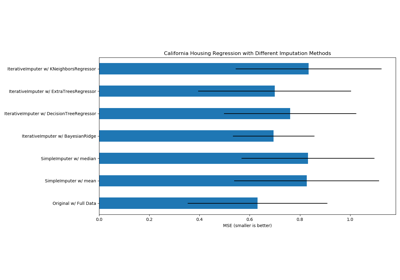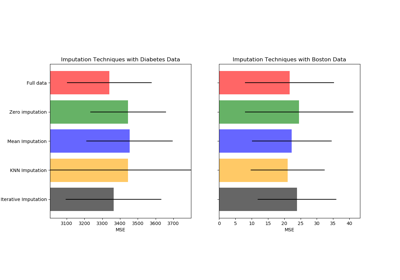# sklearn.impute.IterativeImputer¶

class sklearn.impute.IterativeImputer(estimator=None, missing_values=nan, sample_posterior=False, max_iter=10, tol=0.001, n_nearest_features=None, initial_strategy='mean', imputation_order='ascending', skip_complete=False, min_value=None, max_value=None, verbose=0, random_state=None, add_indicator=False)[source]

Multivariate imputer that estimates each feature from all the others.

A strategy for imputing missing values by modeling each feature with missing values as a function of other features in a round-robin fashion.

Read more in the User Guide.

Note

This estimator is still experimental for now: the predictions and the API might change without any deprecation cycle. To use it, you need to explicitly import enable_iterative_imputer:

>>> # explicitly require this experimental feature
>>> from sklearn.experimental import enable_iterative_imputer  # noqa
>>> # now you can import normally from sklearn.impute
>>> from sklearn.impute import IterativeImputer

Parameters
estimatorestimator object, default=BayesianRidge()

The estimator to use at each step of the round-robin imputation. If sample_posterior is True, the estimator must support return_std in its predict method.

missing_valuesint, np.nan, default=np.nan

The placeholder for the missing values. All occurrences of missing_values will be imputed.

sample_posteriorboolean, default=False

Whether to sample from the (Gaussian) predictive posterior of the fitted estimator for each imputation. Estimator must support return_std in its predict method if set to True. Set to True if using IterativeImputer for multiple imputations.

max_iterint, default=10

Maximum number of imputation rounds to perform before returning the imputations computed during the final round. A round is a single imputation of each feature with missing values. The stopping criterion is met once abs(max(X_t - X_{t-1}))/abs(max(X[known_vals])) < tol, where X_t is X at iteration t. Note that early stopping is only applied if sample_posterior=False.

tolfloat, default=1e-3

Tolerance of the stopping condition.

n_nearest_featuresint, default=None

Number of other features to use to estimate the missing values of each feature column. Nearness between features is measured using the absolute correlation coefficient between each feature pair (after initial imputation). To ensure coverage of features throughout the imputation process, the neighbor features are not necessarily nearest, but are drawn with probability proportional to correlation for each imputed target feature. Can provide significant speed-up when the number of features is huge. If None, all features will be used.

initial_strategystr, default=’mean’

Which strategy to use to initialize the missing values. Same as the strategy parameter in sklearn.impute.SimpleImputer Valid values: {“mean”, “median”, “most_frequent”, or “constant”}.

imputation_orderstr, default=’ascending’

The order in which the features will be imputed. Possible values:

“ascending”

From features with fewest missing values to most.

“descending”

From features with most missing values to fewest.

“roman”

Left to right.

“arabic”

Right to left.

“random”

A random order for each round.

skip_completeboolean, default=False

If True then features with missing values during transform which did not have any missing values during fit will be imputed with the initial imputation method only. Set to True if you have many features with no missing values at both fit and transform time to save compute.

min_valuefloat, default=None

Minimum possible imputed value. Default of None will set minimum to negative infinity.

max_valuefloat, default=None

Maximum possible imputed value. Default of None will set maximum to positive infinity.

verboseint, default=0

Verbosity flag, controls the debug messages that are issued as functions are evaluated. The higher, the more verbose. Can be 0, 1, or 2.

random_stateint, RandomState instance or None, default=None

The seed of the pseudo random number generator to use. Randomizes selection of estimator features if n_nearest_features is not None, the imputation_order if random, and the sampling from posterior if sample_posterior is True. Use an integer for determinism. See the Glossary.

If True, a MissingIndicator transform will stack onto output of the imputer’s transform. This allows a predictive estimator to account for missingness despite imputation. If a feature has no missing values at fit/train time, the feature won’t appear on the missing indicator even if there are missing values at transform/test time.

Attributes
initial_imputer_object of type sklearn.impute.SimpleImputer

Imputer used to initialize the missing values.

imputation_sequence_list of tuples

Each tuple has (feat_idx, neighbor_feat_idx, estimator), where feat_idx is the current feature to be imputed, neighbor_feat_idx is the array of other features used to impute the current feature, and estimator is the trained estimator used for the imputation. Length is self.n_features_with_missing_ * self.n_iter_.

n_iter_int

Number of iteration rounds that occurred. Will be less than self.max_iter if early stopping criterion was reached.

n_features_with_missing_int

Number of features with missing values.

indicator_sklearn.impute.MissingIndicator

Indicator used to add binary indicators for missing values. None if add_indicator is False.

random_state_RandomState instance

RandomState instance that is generated either from a seed, the random number generator or by np.random.

SimpleImputer

Univariate imputation of missing values.

Notes

To support imputation in inductive mode we store each feature’s estimator during the fit phase, and predict without refitting (in order) during the transform phase.

Features which contain all missing values at fit are discarded upon transform.

References

Rcd31b817a31e-1

Stef van Buuren, Karin Groothuis-Oudshoorn (2011). “mice: Multivariate Imputation by Chained Equations in R”. Journal of Statistical Software 45: 1-67.

Rcd31b817a31e-2

S. F. Buck, (1960). “A Method of Estimation of Missing Values in Multivariate Data Suitable for use with an Electronic Computer”. Journal of the Royal Statistical Society 22(2): 302-306.

Examples

>>> import numpy as np
>>> from sklearn.experimental import enable_iterative_imputer
>>> from sklearn.impute import IterativeImputer
>>> imp_mean = IterativeImputer(random_state=0)
>>> imp_mean.fit([[7, 2, 3], [4, np.nan, 6], [10, 5, 9]])
IterativeImputer(random_state=0)
>>> X = [[np.nan, 2, 3], [4, np.nan, 6], [10, np.nan, 9]]
>>> imp_mean.transform(X)
array([[ 6.9584...,  2.       ,  3.        ],
[ 4.       ,  2.6000...,  6.        ],
[10.       ,  4.9999...,  9.        ]])


Methods

 fit(self, X[, y]) Fits the imputer on X and return self. fit_transform(self, X[, y]) Fits the imputer on X and return the transformed X. get_params(self[, deep]) Get parameters for this estimator. set_params(self, \*\*params) Set the parameters of this estimator. transform(self, X) Imputes all missing values in X.
__init__(self, estimator=None, missing_values=nan, sample_posterior=False, max_iter=10, tol=0.001, n_nearest_features=None, initial_strategy='mean', imputation_order='ascending', skip_complete=False, min_value=None, max_value=None, verbose=0, random_state=None, add_indicator=False)[source]

Initialize self. See help(type(self)) for accurate signature.

fit(self, X, y=None)[source]

Fits the imputer on X and return self.

Parameters
Xarray-like, shape (n_samples, n_features)

Input data, where “n_samples” is the number of samples and “n_features” is the number of features.

yignored
Returns
selfobject

Returns self.

fit_transform(self, X, y=None)[source]

Fits the imputer on X and return the transformed X.

Parameters
Xarray-like, shape (n_samples, n_features)

Input data, where “n_samples” is the number of samples and “n_features” is the number of features.

yignored.
Returns
Xtarray-like, shape (n_samples, n_features)

The imputed input data.

get_params(self, deep=True)[source]

Get parameters for this estimator.

Parameters
deepbool, default=True

If True, will return the parameters for this estimator and contained subobjects that are estimators.

Returns
paramsmapping of string to any

Parameter names mapped to their values.

set_params(self, **params)[source]

Set the parameters of this estimator.

The method works on simple estimators as well as on nested objects (such as pipelines). The latter have parameters of the form <component>__<parameter> so that it’s possible to update each component of a nested object.

Parameters
**paramsdict

Estimator parameters.

Returns
selfobject

Estimator instance.

transform(self, X)[source]

Imputes all missing values in X.

Note that this is stochastic, and that if random_state is not fixed, repeated calls, or permuted input, will yield different results.

Parameters
Xarray-like of shape (n_samples, n_features)

The input data to complete.

Returns
Xtarray-like, shape (n_samples, n_features)

The imputed input data.

## Examples using sklearn.impute.IterativeImputer`¶